# Moving Average – an essential technical tool for traders to buy stocks

by Titas Ghosh on
• 11
•
•
•
•
•
•
11
Shares

In technical analysis price is everything. But while trading with the help of technical analysis only price is not enough to understand the market sentiment. To get a better picture of the market traders uses different types of indicators and oscillators like moving average, Relative Strength Index(RSI), Stochastic etc.

In this article, we will discuss moving average indicator. The indicator not only helps a trader to understand the market situation it also helps to take better trading decisions.

There are different types of moving average present in the field of technical analysis let’s discuss one by one.

## Simple Moving Average: –

Simple Moving Average or SMA is a moving average which is calculated by adding the closing price of security prices for the last n-periods and dividing it by the total number of time periods.

For example, suppose we want to calculate the 9 periods SMA of a security price.

First, we will add the last 9 Days closing price of the security and then it will be divided by the 9 periods.

Calculation for 9 periods SMA:

(P9+P8+P7+P6…. +P1)/9

Where,

P=Price

P9= Price 9days ago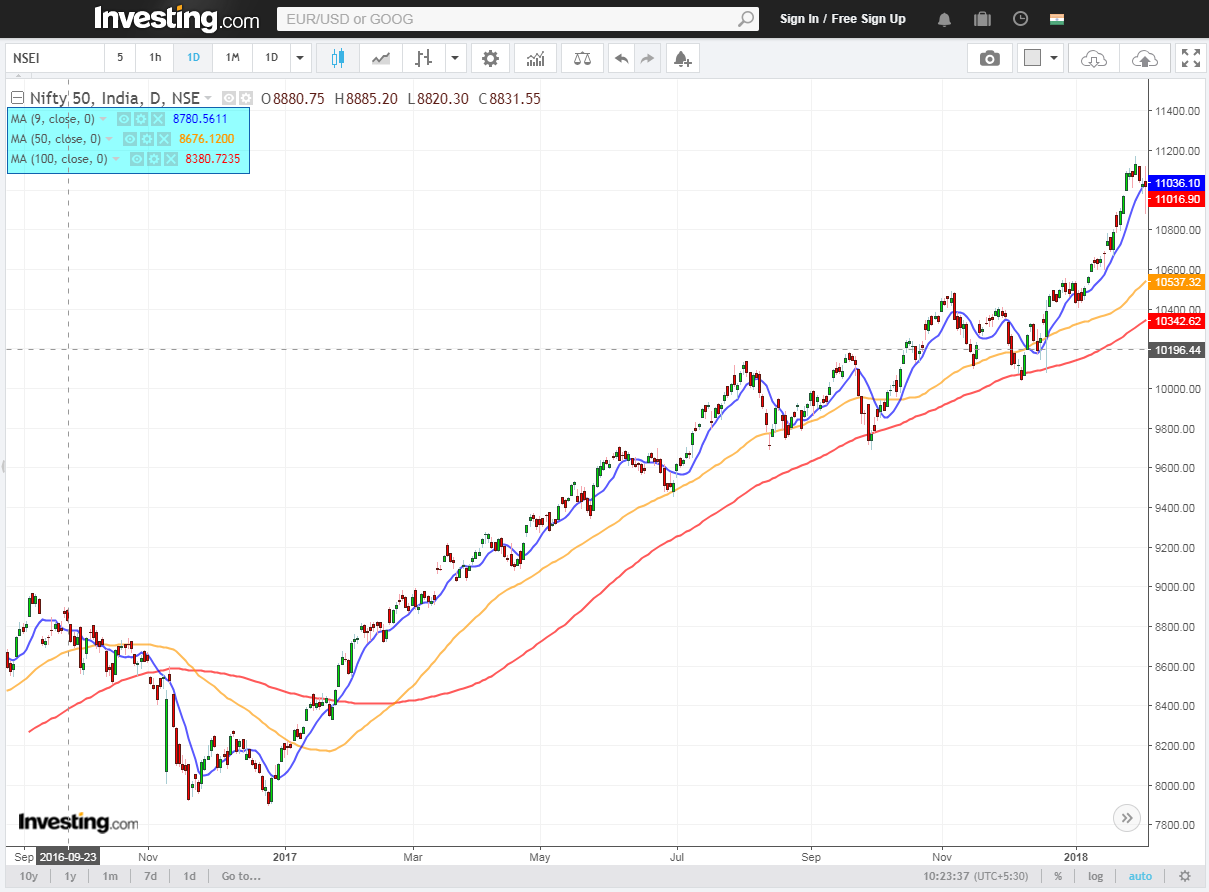SMA is a Technical indicator which is represented by a line and it is directly plotted on the security price. As per the choice of the trader, the periods can be changed in the SMA indicator.

For shorter-term SMA, we can use 5,9,13 etc. For Medium term 20,34,50 and for longer term 100,200 can be used.

## Weighted Moving Average: –

Sometimes technical traders feel that only closing or opening price is not enough for calculating a moving average and this was one of the most important reasons behind the introduction of Weighted Moving Average.

Weighted Moving Average(WMA) concentrate more on recent price action and like SMA it is also plotted directly on the security price.

While calculating SMA, we have seen that equal weightage is given to individual price. But, in case of WMA, it is not the same. Here the latest price gets the maximum importance or weightage.

Suppose we want to calculate the 9 periods WMA of a security price. Then the recent price will get the maximum importance compare to previous prices. In this way, the last day’s price (9 periods ago) will get the minimum weightage.

Calculation of a 9 periods WMA –

WMA = (P1 * 9) + (P2 * 8) + (P3 * 7) +…….+ (P9 * 1) / (9+8+7+6+5 + 4+ 3 + 2 + 1)

where,

P1= Recent price of the security

P2= Price one day ago.

P3= Price two days ago etc.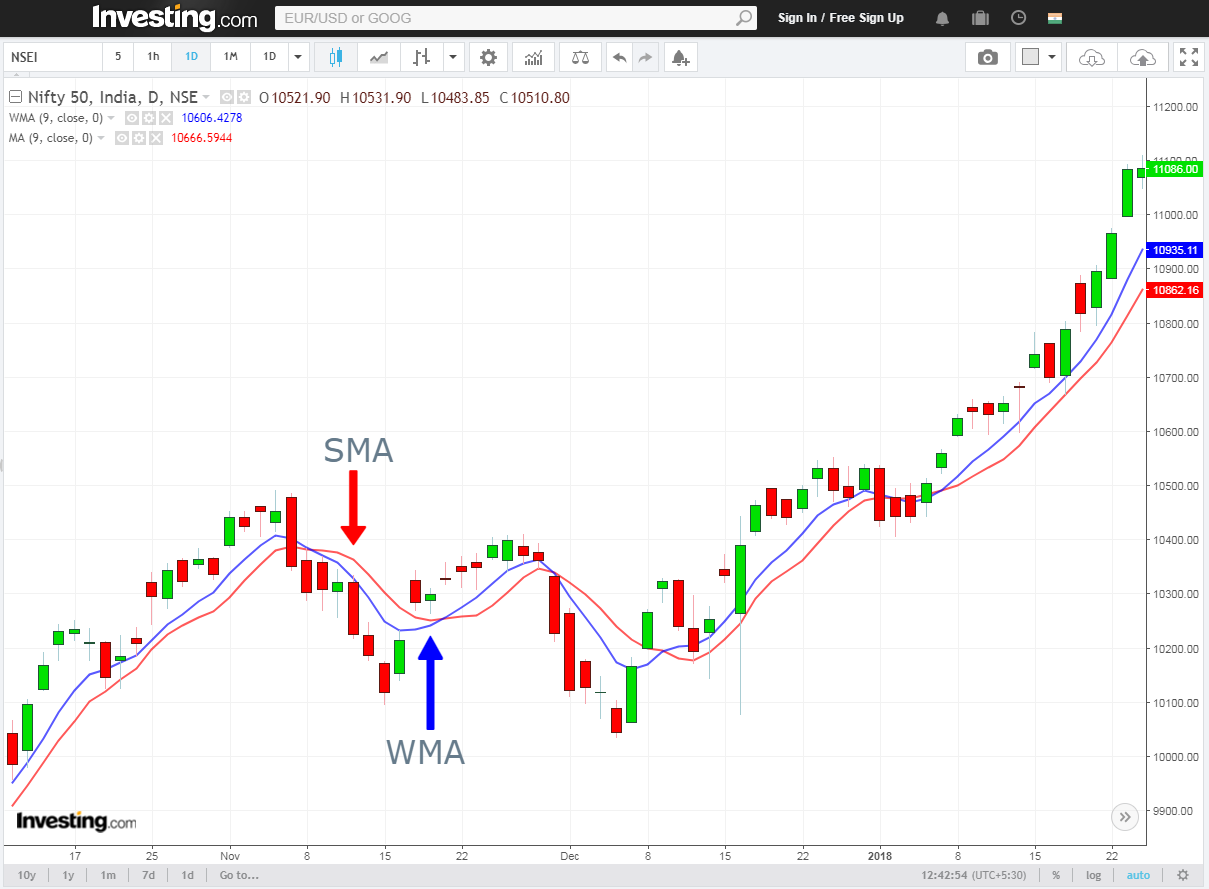## Exponential Moving Average:-

Exponential Moving Average or EMA is quite similar to the WMA. EMA also gives more weight to recent prices. Like other moving averages, EMA is also plotted directly on the prices.

The main difference is in the calculation of this moving average. While calculating the EMA, the decreasing rate of one price to another is not same.  Price decreases exponentially.

There are three steps required to calculate the EMA.

1> Finding out the SMA

2>Calculating the Weighting multiplier

3>Finding out the EMA

For, SMA = (Sum of closing price)/Number of time periods

Weighting multiplier= (2 / (Time period + 1))

and then,

EMA= Closing price-EMA (previous day)) x Weighting multiplier + EMA (previous day)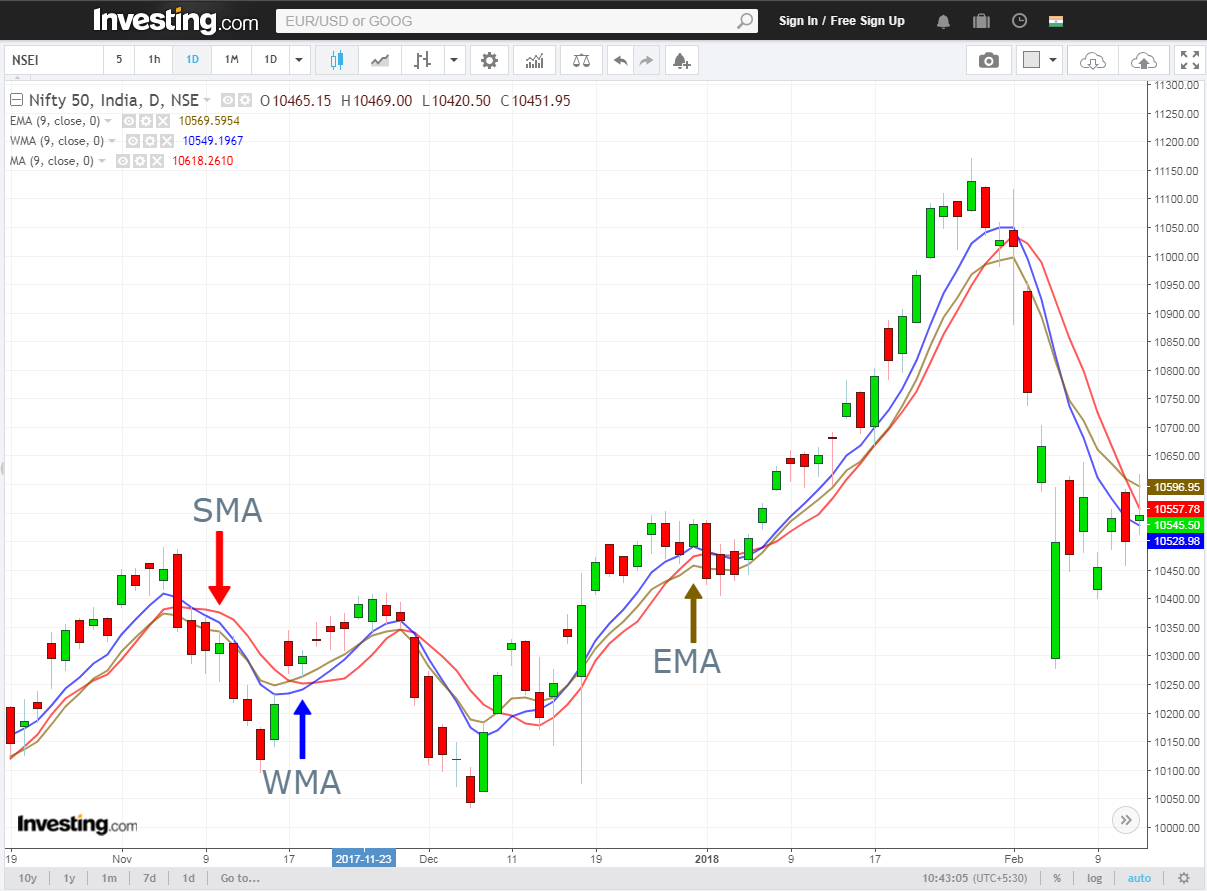Calculating the EMA is a little bit complicated but thanks to the advanced charting system. There is no need to calculate the EMA manually or by your own, EMA is calculated automatically and it is easily available in most of the charting platforms.

## The 5-9 Day Short Term Moving Average Rule:-

5 and 9 EMA both are considered as a short-term moving average. These moving averages follow price more closely relative to a medium or longer-term moving average.

It is pretty much obvious that EMA 5 will fluctuate more than the 9EMA according to the price movement.

This combination of EMAs is very popular among the traders. They help trader by providing early trade signals and good entry, exit points.

Signals occur when short-term EMA (5) crosses the longer-term EMA (9). Depending upon the nature of the crossover Buy and Sell signals generate.

If the EMA 5 crosses the 9 EMA from below it will generate positive crossover signal and according to that, a buy position can be taken. Just opposite happens when the 5 EMA crosses the 9 EMA from above. This crossover named as a negative crossover and depending on that signal a trader can take a short position.

Learn how to trade using a moving average crossover strategy: 5, 30 Moving Average crossover strategy using ROC

below are few examples of positive a negative crossover.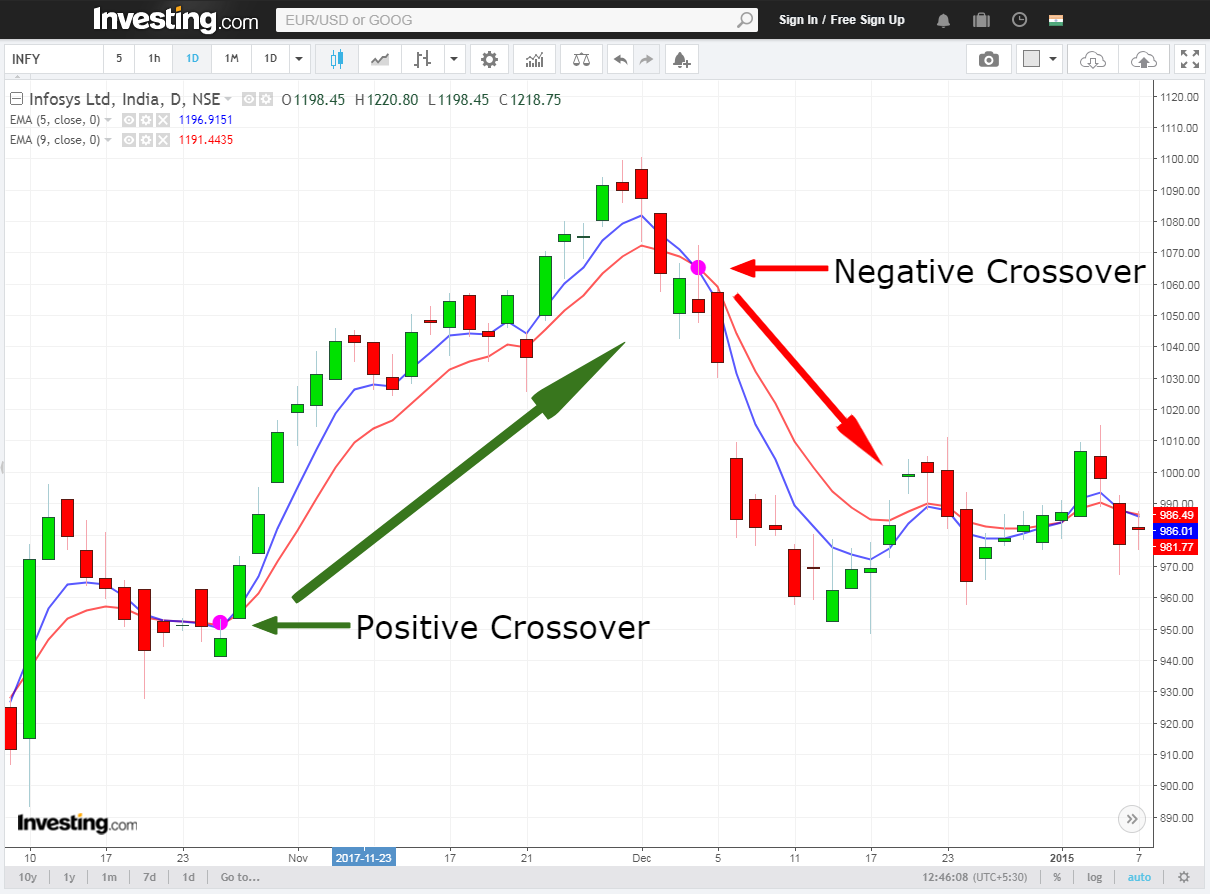This one-day chart of Infosys showing a positive and negative crossover.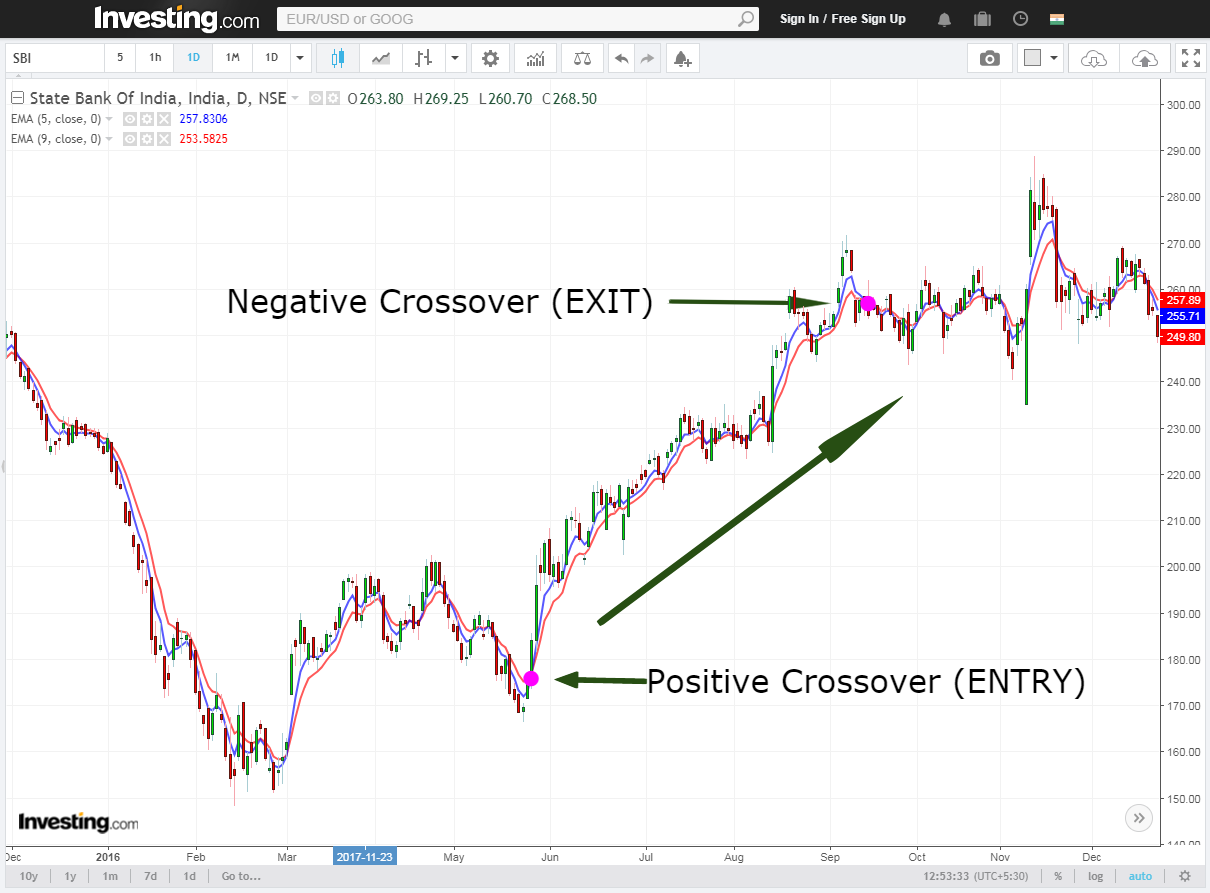This is the daily chart of SBI. A positive crossover happened during the month of May-June and the stock moved nearly 80points in the upward direction.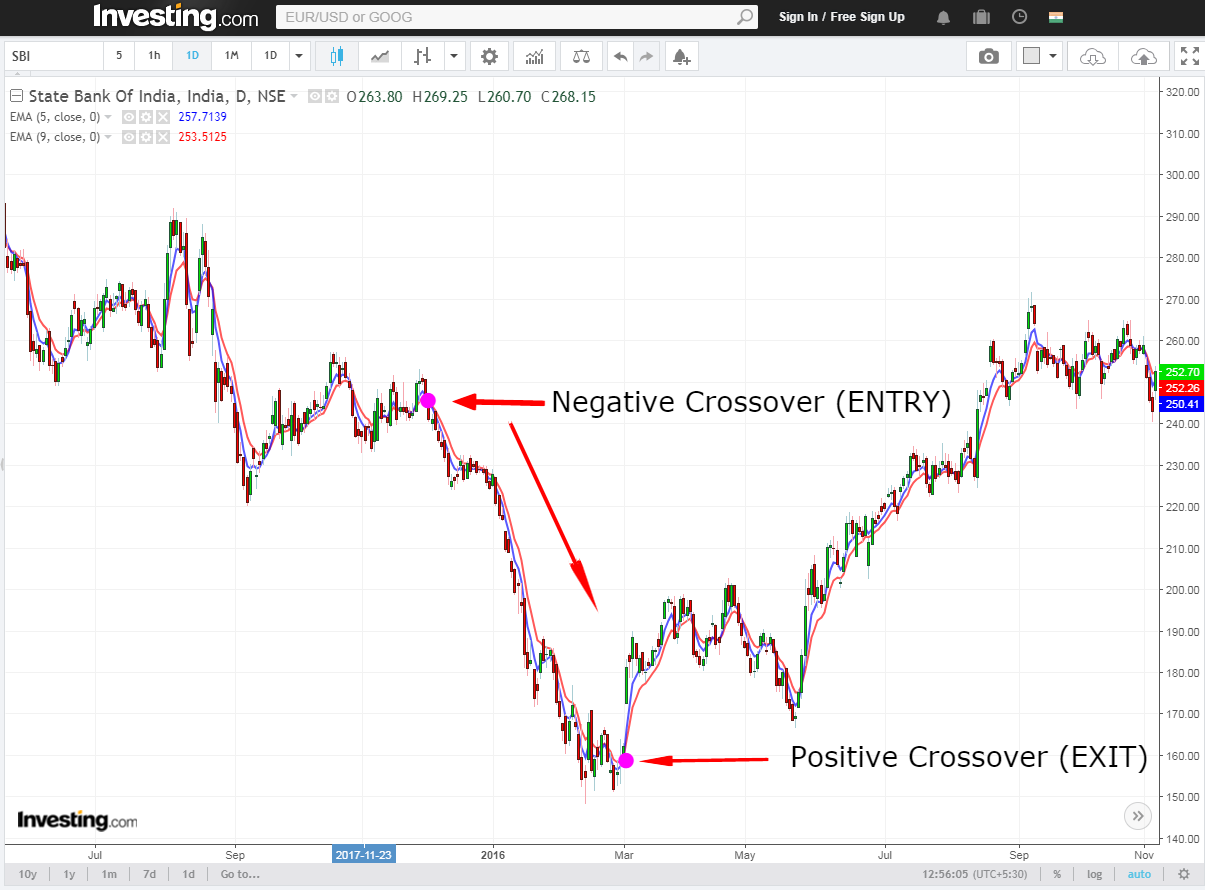Another example also from SBI. This negative crossover happened in the daily chart. This time also it gave nearly 80 points downward move.

Drawbacks:

1>Due to the high fluctuation of these EMAs they often end up generating false trade signals.

2> Does not work well in choppy market condition.

3> Signals generates very frequently.

It is advisable that do not trade only using these two EMAs. Take the other indicators like RSI and stochastics for better trading decisions.

You can learn more about technical tools, indicators, oscillators, technical charts etc. you can enroll for  NSE Academy Certified Technical Analysis

## How do 50-day, 100-day, and 200-day moving averages differ:-

These are the most important SMAs in technical analysis. SMA 50 is considered as a medium-term moving average SMA but 100 SMA and 200 SMA both are considered as long-term moving average.

The main difference lies in the calculation of these SMAs. SMA 50 requires 50 time periods to calculate, in case of 100 MA and 200 MA 100time periods and 200 time periods are required.

For short-term trades, 50SMA plays an important role and it is considered as a basic resistance and support line. In longer-term time frame, 100 SMA and 200SMAs both play the same role but weightage of these lines are greater than 50 SMA line. It is always better to take a trade at these levels because the price in these level is generally less risky to enter because they are good support levels.

Read more about Support and Resistance: A comprehensive study on Support and Resistance

For SMA 50 it is constructed by using the 50 trading day’s closing price. So basically traders see it as a line which is representing 10weeks’ average closing price. Similarly, for SMA 100 line and SMA 200 line represents the average closing price of 20 weeks and 40 weeks.## Bottom-line:

Moving averages are very useful indicators. They suggest good entry and exit points while trading. The simplicity of this indicator makes it popular among the traders. But it has some drawbacks too. In a highly volatile market condition, short-term MAs generate false trade signals which lead to a loss. Moving Average does not work well in choppy market condition and it is advisable to not to use shorter or medium term MAs during that period.

Every indicator has different drawbacks but it is also true that they work well if they are combined with other indicators and oscillators. RSI and Stochastics are one of the most commonly used indicators and oscillator to combine with a moving average.

• 11
•
•
•
•
•
•
11
Shares

Disclaimer

Elearnmarkets.com wants to remind you that all our content is created solely for the purpose of education. No strategy, stock, commodity, fund or any other security discussed here is any way a recommendation for trading or investing. Elearnmarkets.com will not be any way responsible for trading losses incurred by any individual or entity for trading with real money. Please take advise of certified financial advisers before trading or investing.

This site uses Akismet to reduce spam. Learn how your comment data is processed.